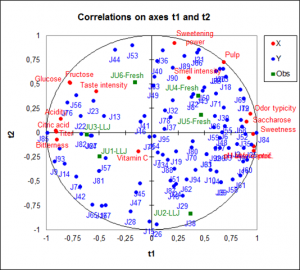# Partial Least Squares regression (PLS)

Partial Least Squares regression (PLS) is often used when there are a lot of explanatory variables, possibly correlated. Available in Excel with XLSTAT.## Partial Least Squares Regression (PLS)

Partial Least Squares regression (PLS) is a quick, efficient and optimal regression method based on covariance. It is recommended in cases of regression where the number of explanatory variables is high, and where it is likely that there is multicollinearity among the variables, i.e. that the explanatory variables are correlated.

XLSTAT provides a complete PLS regression method to model and predict your data in excel. XLSTAT proposes several standard and advanced options that will let you gain a deep insight on your data:

• Choose several response variables in one analysis
• Use the leave one out (LOO) cross validation option
• Automatically choose the number of components to be kept using one of multiple criteria or choose this number manually
• Choose between the fast algorithm and the more precise one.

## What is Partial Least Squares regression?

The Partial Least Squares regression (PLS) is a method which reduces the variables, used to predict, to a smaller set of predictors. These predictors are then used to perfom a regression.

The idea behind the PLS regression is to create, starting from a table with n observations described by p variables, a set of h components with the PLS 1 and PLS 2 algorithms

Some programs differentiate PLS 1 from PLS 2. PLS 1 corresponds to the case where there is only one dependent variable. PLS 2 corresponds to the case where there are several dependent variables. The algorithms used by XLSTAT are such that the PLS 1 is only a particular case of PLS 2.

## Partial Least Squares regression model equations

In the case of the Ordinary Least Squares (OLS) and Principale Component Regression (PCR) methods, if models need to be computed for several dependent variables, the computation of the models is simply a loop on the columns of the dependent variables table Y. In the case of PLS regression, the covariance structure of Y also influences the computations.

The equation of the PLS regression model writes:

Y = ThC’h + Eh = XWh*C’h + Eh = XWh (P’hWh)-1 C’h + Eh

where Y is the matrix of the dependent variables, X is the matrix of the explanatory variables. Th, Ch, W*h , Wh and Ph, are the matrices generated by the PLS algorithm, and Eh is the matrix of the residuals.

The matrix B of the regression coefficients of Y on X, with h components generated by the PLS regression algorithm is given by:

B = Wh(P’hWh)-1C’hNote: the PLS regression leads to a linear model as the OLS and PCR do.

PLS regression results: Correlation, observations charts and biplots

A great advantage of PLS regression over classic regression are the available charts that describe the data structure. Thanks to the correlation and loading plots it is easy to study the relationship among the variables. It can be relationships among the explanatory variables or dependent variables, as well as between explanatory and dependent variables. The score plot gives information about sample proximity and dataset structure. The biplot gather all these information in one chart.

## Prediction with Partial Least Squares regression

PLS regression is also used to build predictive models. XLSTAT enables you to predict new samples' values.

## General remarks about PLS regression

The three methods – Partial Least Squares regression (PLS), Principal Component regression (PCR), which is based on Principal Component analysis (PCA),  and Ordinary Least Squares regression (OLS), which is the regular linear regression,  - give the same results if the number of components obtained from the Principal Component analysis (PCA) in the PCR, or from the PLS regression is equal to the number of explanatory variables.

### What is the difference between PCR and PLS regression?

The components obtained from the PLS regression,which is based on covariance, are built so that they explain as well as possible Y, while the components of the PCR are built to describe X as well as possible. This explains why the PLS regression outperforms PCR when the target is strongly correlated with a direction in the data that have a low variance. The XLSTAT-PLS software allows partly compensating this drawback of the PCR by allowing the selection of the components that are the most correlated with Y.

Here is an example on how to run a Partial Least Squares regression (PLS).### analyze your data with xlstat

14-day free trial

Included in

Related features